# MP : Quiz d’apprentissage

## Intégration

MP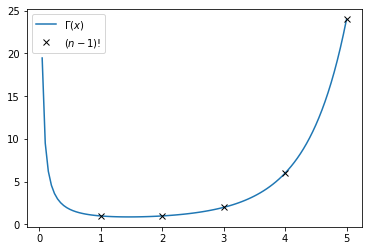Intégration

Des questions sur les intégrales sur un intervalle quelconque et les intégrales à paramètres.

10 questions prises au hasard dans la base.

1 / 10

Si (f_n) est une suite de fonctions continues sur un intervalle I qui converge uniformément vers f sur I, alors les les intégrales sur I de f_n tendent vers l'intégrale sur I de f.

2 / 10

Si f continue par morceaux est bornée sur un intervalle I, alors est intégrable sur I.

3 / 10

Soit I un intervalle. Une fonction constante sur I est intégrable.

4 / 10

Si f est intégrable sur R+, alors f tend vers 0 en l'infini.

5 / 10

Pour x réel strictement positif, Gamma(x) est égal à l'intégrale sur R+ de :

6 / 10

Si f est continue par morceaux sur un segment I, alors elle est intégrable sur I.

7 / 10

L'intégrale sur R+ sur sinus cardinal est

8 / 10

La fonction définie par f(t) = exp(-t) est intégrable

9 / 10

Si f est continue et positive sur un intervalle I, alors elle est intégrable sur I.

10 / 10

La fonction Gamma est

Votre note est de

The average score is 40%

0%

## Probabilités

MP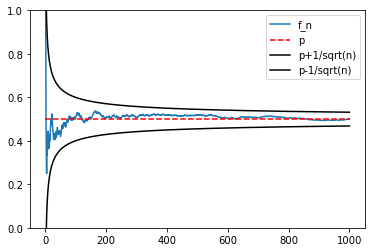Probabilités

Des questions sur les probabilités et les variables aléatoires discrètes.

10 questions prises au hasard dans la base.

Si E[X] = 9 et V(X) = 4, alors la variable aléatoire centrée réduite associée à X est

On lance une pièce à Pile ou Face jusqu'à obtenir un Pile et on note X le nombre de tirages effectués. Alors X suit

Si X et Y sont deux variables aléatoires indépendantes de loi de Poisson de paramètre 1, alors X+Y

Si X_1,..., X_n sont des variables aléatoires indépendantes de loi B(p), alors la somme des X_i suit une loi...

Si X est à valeurs dans N* et telle que, pour tout n >0, P(X=n) > 1/n², alors

Si on lance un dé équilibré à six faces un grand nombre de fois, alors le score moyen est environ égal à ...

Si X suit une loi géométrique de paramètre p, alors son univers-image est

Si X admet une variance finie, alors E[X²] =

Si X et Y sont deux variables aléatoires admettant une variance finie, alors

On lance une pièce équilibrée jusqu'à obtenir Pile. En moyenne, on la lancera:

Votre note est de

The average score is 56%

0%

## Algèbre

MP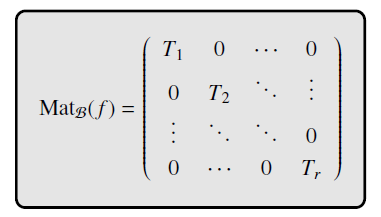Réduction

Des questions sur la réduction des matrices et des endomorphismes.

10 questions prises au hasard dans la base.

A est diagonalisable si, et seulement si, son polynôme caractéristique est scindé à racines simples.

Si f est diagonalisable et F est un sev stable par f, alors la restriction de f à F est diagonalisable

Si A est une matrice telle que A² = 0, alors (A+I)^n =

Si A est une matrice nilpotente, sa seule valeur propre possible est 0.

Si 0 est une valeur propre de A, alors :

Toute matrice de M(n,R) est

Une matrice de taille n admet au plus n valeurs propres.

Si A est une matrice de taille 3 admettant uniquement 2 valeurs propres alors elle n'est pas diagonalisable.

Si une matrice de M(n,K) admet n valeurs propres distinctes, alors:

Une matrice quelconque admet au moins une valeur propre.

Votre note est de

The average score is 66%

0%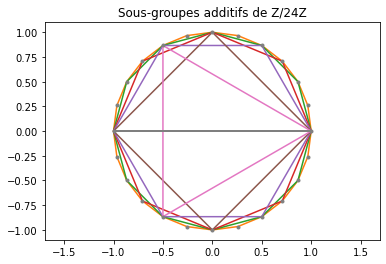Algèbre générale

1 / 10

Le groupe U(Z/5Z) des inversibles de Z/5Z contient...

2 / 10

Le groupe symétrique S_3 est isomorphe à un sous-groupe de S_n dès que n est supérieur ou égal à 3.

3 / 10

L'ensemble des matrices de déterminant nul est un groupe pour le produit matriciel.

4 / 10

(Z/6Z,+,*) est...

5 / 10

Parmi les applications suivantes, lesquelles sont des morphismes de groupes définis sur GL(n,K) muni du produit matriciel ?

6 / 10

Z/9Z est un groupe isomorphe à (Z/3Z)^2

7 / 10

(Z/6Z,+,*) contient exactement...

8 / 10

33 est inversible dans (Z/121Z,+,*)

9 / 10

Le groupe symétrique sur n éléments contient...

10 / 10

Si G est un groupe monogène de cardinal premier, alors tout élément non trivial de G est un générateur de G.

Votre note est de

The average score is 52%

0%

## Suites et séries

15

MP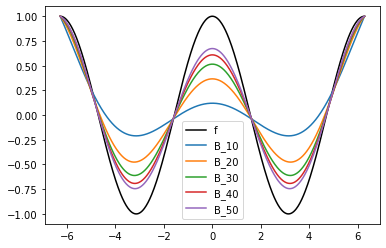Suites, séries de fonctions et séries entières

10 questions au hasard sur le programme de MP concernant les suites, séries de fonctions et les séries entières.

1 / 10

Si on note R le rayon de convergence d'une série entière et R' celui de sa dérivée, on a :

2 / 10

Soit R_1 le rayon de convergence de \sum (x^n)/n et R_2 celui de \sum (x^n)/n^2. Alors

3 / 10

La série de terme général (f_n) converge uniformément si et seulement si...

4 / 10

Pour qu'une fonction soit développable en série entière il [texte à compléter] qu'elle soit de classe C infini

5 / 10

Si une série de fonctions converge normalement sur I, alors

6 / 10

Si a(n) est équivalent à 1/n quand n tend vers l'infini, alors le rayon de convergence de la série entière \sum a_n z_n vérifie

7 / 10

Sur le bord du disque de convergence, une série entière peut

8 / 10

Si la série entière \sum a_n z^n a pour rayon de convergence R>0, alors :

9 / 10

Toute fonction continue sur un intervalle I est limite uniforme d'une suite de polynômes.

10 / 10

Si (f_n) converge simplement vers f sur [a,b], alors la suite des intégrales de f_n sur [a,b] est égale à l'intégrale de f sur [a,b].

Votre note est de

The average score is 28%

0%
31

MP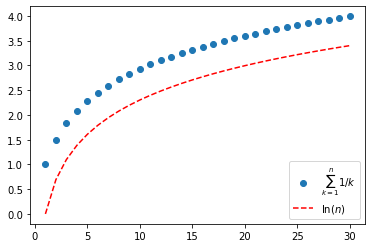Séries numériques

Quiz d'apprentissage sur les séries numériques en MP

10 questions prises au hasard dans la base

1 / 10

Si |x|<1, la série de terme général x^n, pour n entier naturel,...

2 / 10

Soient (u_n) et (v_n) des suites à termes positifs. On suppose que les séries correspondantes convergent et on note respectivement U et V leurs sommes. Alors la série de terme général u_n*v_n converge et la somme de cette série est UV.

3 / 10

Si (u_n) converge vers 1. Que peut-on dire de la série \sum (u_n-u_{n+1}) ?

4 / 10

Si (u_n) est une suite à termes strictement positifs telle que u_{n+1}/u_n tend vers 0. Alors

5 / 10

La série de terme général (-1)^n/sqrt(n) est

6 / 10

La série de terme général (-1)^n/n!, pour n entier naturel,...

7 / 10

La série de terme général sin(2/n²) est

8 / 10

Si (u_n) et (v_n) sont des suites complexes telles que les séries \sum u_n et \sum v_n convergent absolument. Alors \sum (u_n*v_n) converge absolument.

9 / 10

La série de terme général 1/(n²*ln(n)) est

10 / 10

Pour que la série de terme général u_n converge...

Votre note est de

The average score is 24%

0%